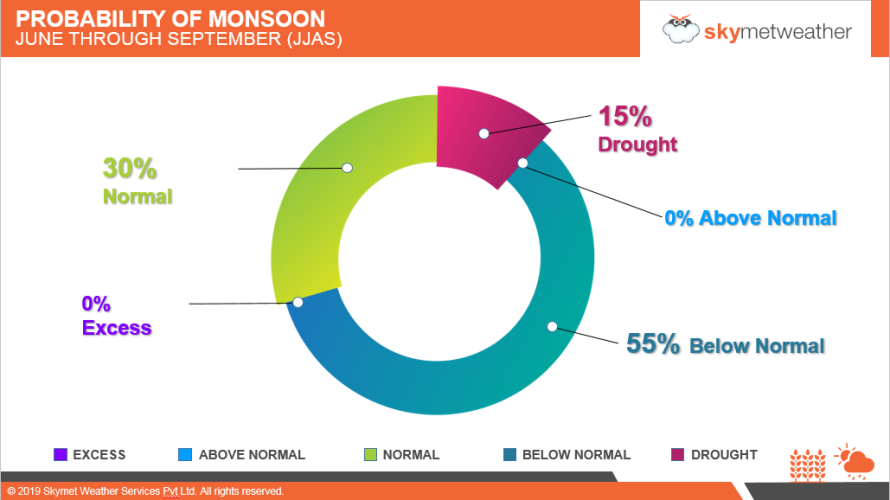# Skymet forecasts below normal Southwest Monsoon in 2019 at 93 percent of LPA

April 3, 2019 1:50 PM |

According to Skymet Weather, Monsoon 2019 is likely to be ‘below normal’ to the tune of 93% (with an error margin of +/-5%) of the long period average (LPA) of 887 mm for the four-month period from June to September.

According to Skymet, Monsoon probabilities for JJAS are:

• 0% chance of excess (seasonal rainfall that is more than 110% of LPA)
• 0% chance of above normal (seasonal rainfall that is between 105 to 110% of LPA)
• 30% chance of normal (seasonal rainfall that is between 96 to 104% of LPA)
• 55% chance of below normal (seasonal rainfall that is between 90 to 95% of LPA)
• 15% chance of drought (seasonal rainfall that is less than 90% of LPA)On a monthly scale, the precipitation foreshadow is as follows:

June – 77% of LPA (LPA for June = 164 mm)

• 15% chance of normal
• 10% chance of above normal
• 75% chance of below normal

July – 91% of LPA (LPA for July= 289 mm)

• 35% chance of normal
• 10% chance of above normal
• 55% chance of below normal

August – 102% of LPA (LPA for August = 261 mm)

• 55% chance of normal
• 15% chance of above normal
• 30% chance of below normal

September – 99% of LPA (LPA for September = 173 mm)

• 55% chance of normal
• 15% chance of above normal
• 30% chance of below normal